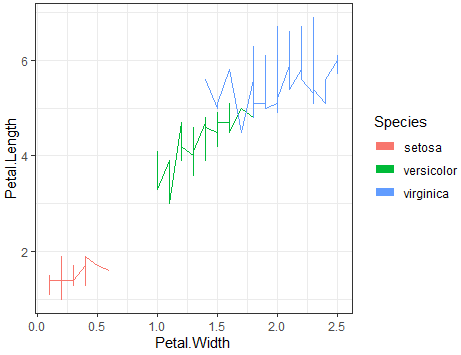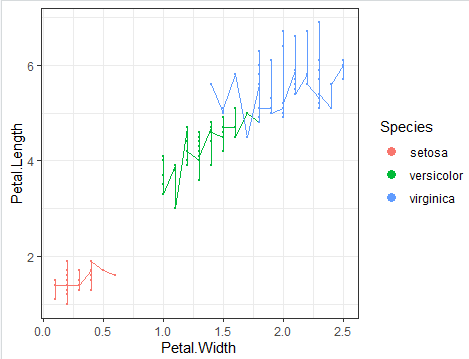3 views

I'm plotting lines with ggplot2 like this:

ggplot(iris, aes(Petal.Width,Petal.Length,color=Species)) + geom_line() + theme_bw()I find legend marks to be small so I want them to be bigger. If I change the size, lines on the plot change too:

ggplot(iris, aes(Petal.Width,Petal.Length,color=Species)) + geom_line(size=4) + theme_bw()But I only want to see thick lines in the legend, I want lines on the plot to be thin. I tried to use legend.key.size but it changes the square of the mark, not the width of the line:

library(grid)  # for unit

ggplot(iris,aes(Petal.Width,Petal.Length,color=Species))+geom_line()+theme_bw() + theme(legend.key.size=unit(1,"cm"))I also tried to use points:

ggplot(iris,aes(Petal.Width,Petal.Length,color=Species)) + geom_line() + geom_point(size=4) + theme_bw()

But of course it still affects both plot and legend:I wanted to use lines for the plot and dots/points for the legend.

How to change the width of the line in the legend without changing the plot?

How to draw lines in the plot, but draw points/dots/squares in the legend?

by

You can use the color argument of the guides function as follows:

ggplot(iris,aes(Petal.Width,Petal.Length,color=Species))+geom_line()+theme_bw()+

guides(colour = guide_legend(override.aes = list(size=3)))

Output:To get points in legend and lines in plot add geom_point(size=0) to make the points invisible and then in guides() set linetype=0 to remove lines and size=3 to get larger points.i.e.,

ggplot(iris,aes(Petal.Width,Petal.Length,color=Species))+geom_line()+theme_bw()+

geom_point(size=0)+

guides(colour = guide_legend(override.aes = list(size=3,linetype=0)))

Output: